# 1. A 10 kg box is at rest at the end of an unstretched spring with...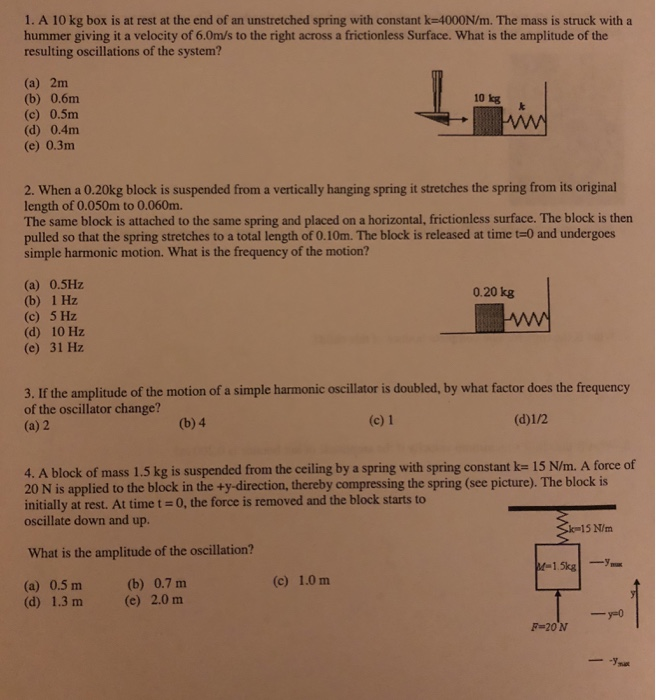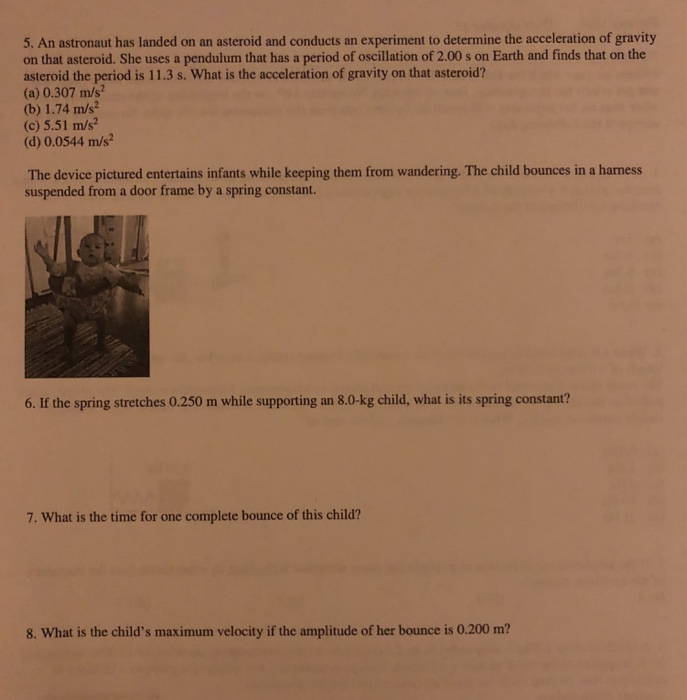1. A 10 kg box is at rest at the end of an unstretched spring with constant k-4000N/m. The mass is struck with a hummer giving it a velocity of 6.0m/s to the right across a frictionless Surface. What is the amplitude of the resulting oscillations of the system? (a) 2m (b) 0.6m (c) 0.5m (d) 0.4m (e) 0.3m 10 kg 2. When a 0.20kg block is suspended from a vertically hanging spring it stretches the spring from its original length of 0.050m to 0.060m. The same block is attached to the same spring and placed on a horizontal, frictionless surface. The block is then pulled so that the spring stretches to a total length of 0.10m. The block is released at time t-0 and undergoes simple harmonic motion. What is the frequency of the motion? (a) 0.5Hz (b) 1 Hz (c) 5 Hz (d) 10 Hz (e) 31 Hz 0.20 kg 3. If the amplitude of the motion of a simple harmonic oscillator is doubled, by what factor does the frequency of the oscillator change? (a) 2 (b) 4 (c) 1 4. A block of mass 1.5 kg is suspended from the ceiling by a spring with spring constant k- 15 N/m. A force of 20 N is applied to the block in the +y-direction, thereby compressing the spring (see picture). The block is initially at rest. At time t 0, the force is removed and the block starts to oscillate down and up. 15 N/m What is the amplitude of the oscillation? (c) 1.0 m (a) 0.5m (d) 1.3 m (b) 0.7 m (e) 2.0 m F 20 N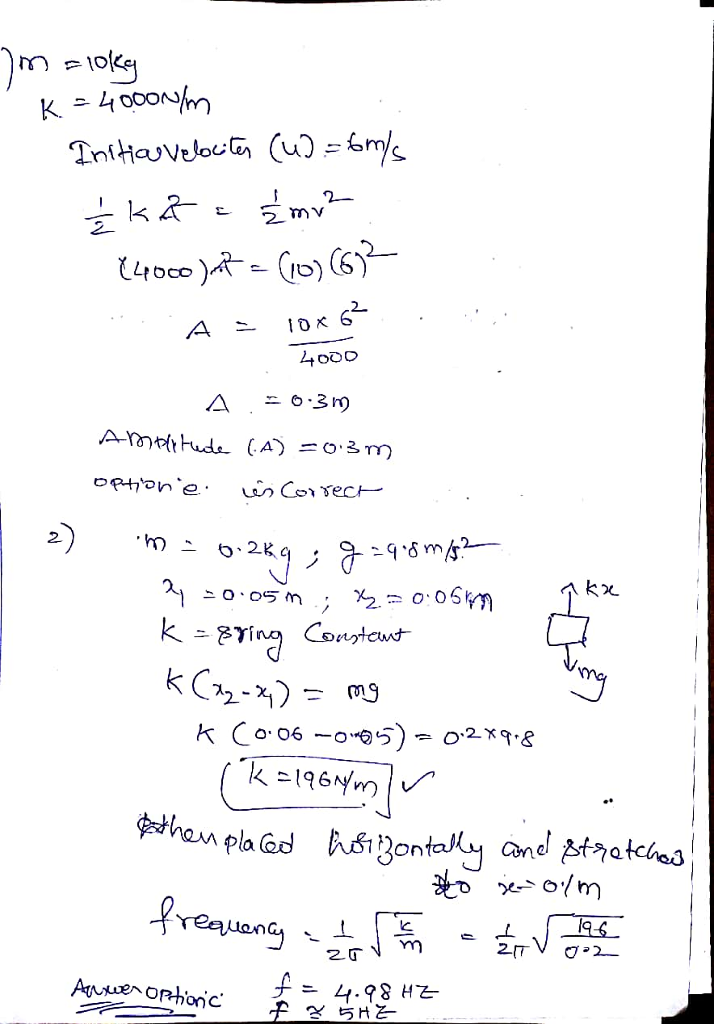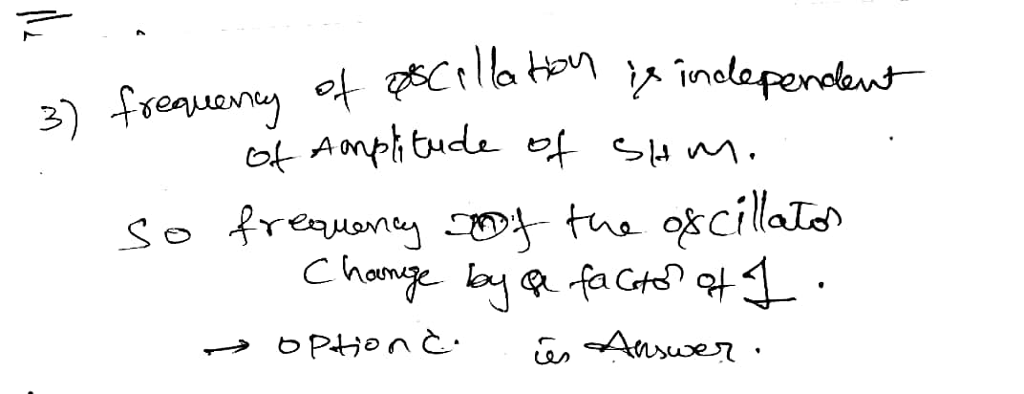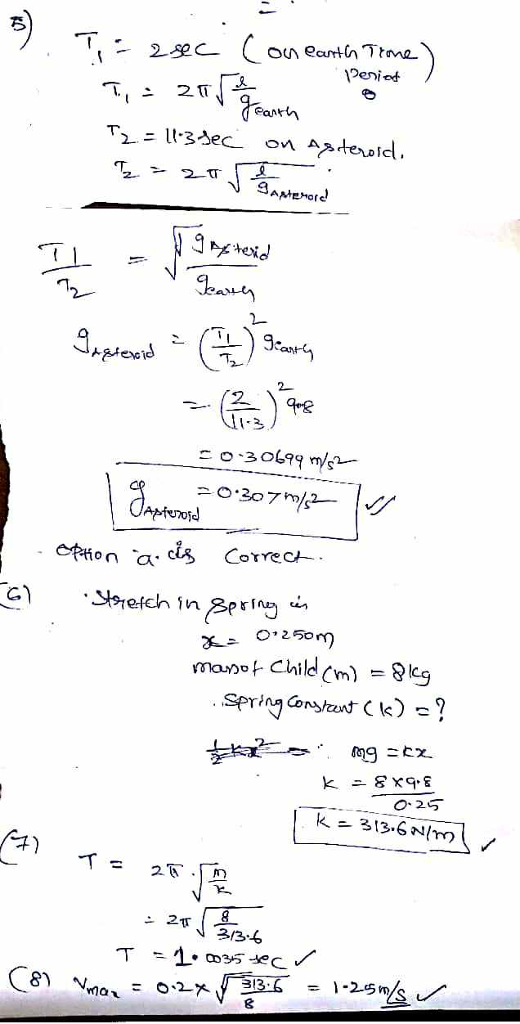#### Earn Coin

Coins can be redeemed for fabulous gifts.

Similar Homework Help Questions
• ### A horizontal mass-spring system consists of a block (m=1.5 kg) on a frictionless to connected to...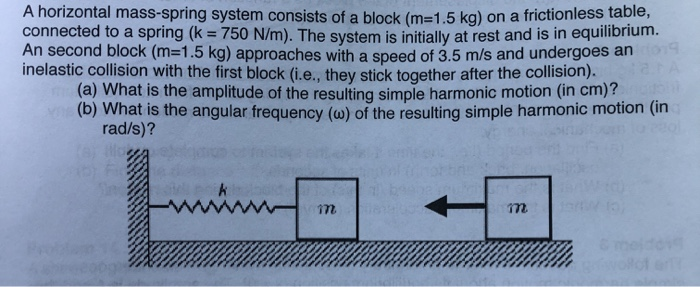A horizontal mass-spring system consists of a block (m=1.5 kg) on a frictionless to connected to a spring (k = 750 N/m). The system is initially at rest and is in equilibrium MI Second DIOCK (M=1.5 kg) approaches with a speed of 3.5 m/s and undergoes all inelastic collision with the first block (i.e.. they stick together after the collision). (a) What is the amplitude of the resulting simple harmonic motion (in cm)? (b) What is the angular frequency (w)...

• ### Homework: Simple Harmonic Motion Name: 1. A 4.5-kg block is hung from a spring causing the...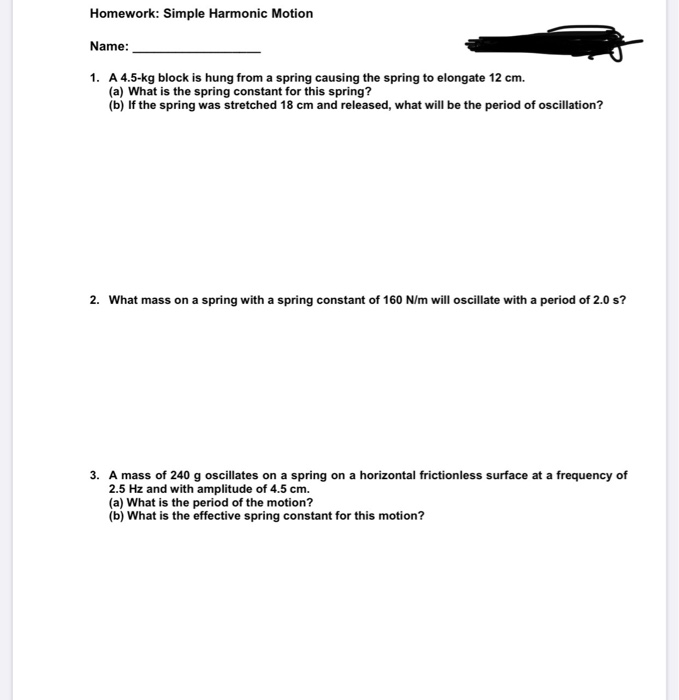Homework: Simple Harmonic Motion Name: 1. A 4.5-kg block is hung from a spring causing the spring to elongate 12 cm. (a) What is the spring constant for this spring? (b) If the spring was stretched 18 cm and released, what will be the period of oscillation? 2. What mass on a spring with a spring constant of 160 N/m will oscillate with a period of 2.0 s? 3. A mass of 240 g oscillates on a spring on a...

• ### A wooden block (m = 0.640 kg) is connected to a spring and undergoes simple harmonic...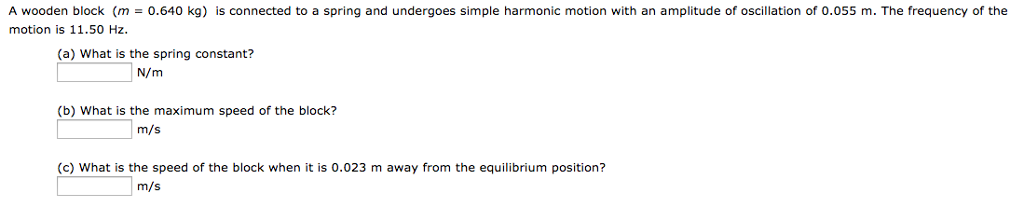A wooden block (m = 0.640 kg) is connected to a spring and undergoes simple harmonic motion with an amplitude of oscillation of 0.055 m. The frequency of the motion is 11.50 Hz. (a) What is the spring constant? N/m (b) What is the maximum speed of the block? m/s (c) What is the speed of the block when it is 0.023 m away from the equilibrium position? m/s

• ### 1) A 12.3 kg particle is undergoing simple harmonic motion with an amplitude of 1.86 mm....

1) A 12.3 kg particle is undergoing simple harmonic motion with an amplitude of 1.86 mm. The maximum acceleration experienced by the particle is 7.93 km/s2. (a) Find the period of the motion. (b) What is the maximum speed of the particle? (c) Calculate the total mechanical energy of this simple harmonic oscillator. 2) The orbit of the Moon around the Earth as projected along a diameter can be viewed as simple harmonic motion. Calculate the effective force constant k...

• ### A block of mass M = 5.60 kg, at rest on a horizontal frictionless table, is...

A block of mass M = 5.60 kg, at rest on a horizontal frictionless table, is attached to a rigid support by a spring of constant k = 6390 N/m. A bullet of mass m = 8.20 g and velocity of magnitude 710 m/s strikes and is embedded in the block (the figure). Assuming the compression of the spring is negligible until the bullet is embedded, determine (a) the speed of the block immediately after the collision and (b) the...

• ### A block of mass M = 1.94 kg, at rest on a horizontal frictionless table, is...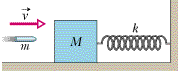A block of mass M = 1.94 kg, at rest on a horizontal frictionless table, is attached to a rigid support by a spring of constant k = 110 N/m. A bullet of mass m = 4.7 g and velocity of magnitude 810 m/s strikes and is embedded in the block (Fig. See below). Assuming the compression of the spring is negligible until the bullet is embedded, determine (a) the speed of the block immediately after the collision and (b)...

• ### A simple harmonic oscillator consists of a block attached to a spring, moving back and forth...

A simple harmonic oscillator consists of a block attached to a spring, moving back and forth on a frictionless horizontal surface. Suppose the mass of the box is 5.0 kg. The motion is started by holding the box at .50m from its central position, using a force of 40.0 N. Then the box is let go and allowed to perform simple harmonic motion. What is the amplitude of the motion? What is the spring constant k? What is the maximum...

• ### A 2-kg object is suspended at rest from a vertical spring (K=196 N/m) attached to the...

A 2-kg object is suspended at rest from a vertical spring (K=196 N/m) attached to the ceiling. From this equilibrium position, the object is pulled down an additional distance d=3 cm and released from rest. a) Considering the upward direction to be positive, find the amplitude, frequency and phase constant of the simple harmonic motion and write the equation of the motion. b) find the speed of the object at the moment when it is 3 cm above the release...

• ### A block of mass 1.20 kg is attached to a horizontal spring that has force constant...

A block of mass 1.20 kg is attached to a horizontal spring that has force constant k = 300 N/m. The block moves on a horizontal frictionless surface. The maximum speed of the block during its motion is 5 m/s. What is the amplitude A of the simple harmonic motion of the block?

• ### A simple harmonic oscillator consists of a block attached to a spring, moving back and forth...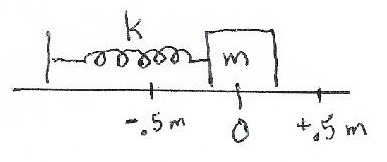A simple harmonic oscillator consists of a block attached to a spring, moving back and forth on a frictionless horizontal surface. Suppose the mass of the box is 5.0 kg. The motion is started by holding the box at 0.50 m from its central position, using a force of 40.0 N. Then the box is let go and allowed to perform simple harmonic motion. (a) What is the amplitude of the motion? (b) What is the spring constant k? (c)...